Parents and Teachers: Support Ducksters by following us onor.

# Kids Math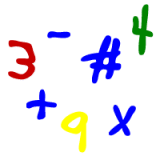Quadrilaterals are four sided polygons. Some quadrilaterals are probably quite familiar to you like squares and rectangles. A unique property of quadrilaterals is that the sum of all their interior angles always totals 360 degrees.

Rectangle

A rectangle is a quadrilateral where all four angles are 90 degrees. A square is a special type of rectangle where all four sides are the same length.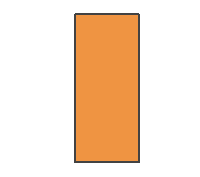Rectangle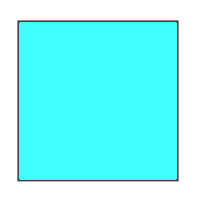Square

Parallelogram

A parallelogram is when both opposing pairs of sides on a quadrilateral are parallel. Squares and rectangles are also parallelograms.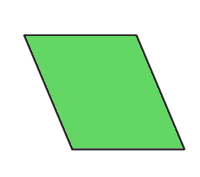Rhombus

A rhombus is a quadrilateral where all four sides have the same length. A square is a rhombus but not all rhombuses are squares.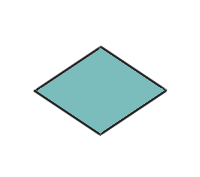Trapezoid

A trapezoid is when there is only one pair of opposing parallel sides (see the picture below). An isosceles trapezoid is a trapezoid where the non-parallel sides are of equal length and it has a line of symmetry down the middle.More Geometry Subjects

Circle
Polygons
Triangles
Pythagorean Theorem
Perimeter
Slope
Surface Area
Volume of a Box or Cube
Volume and Surface Area of a Sphere
Volume and Surface Area of a Cylinder
Volume and Surface Area of a Cone
Angles glossary
Figures and Shapes glossary

Back to Kids Math

Back to Kids StudyFollow us onor